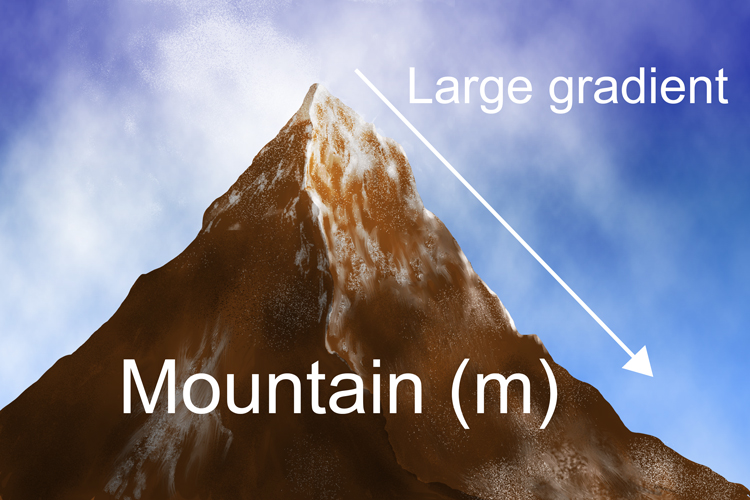# Remember the formula for the gradient

Gradient (m)=(y(chang\e\ i\n))/(x(chang\e\ i\n)

You can remember the formula for the gradient as follows:There is a large gradient on a mountain (m).Put your friend's Y fronts over the eggs (y over x) to stop the eggs from cracking if you're going to roll your eggs down a mountain.

Large gradient is a mountain = m

Y fronts over the eggs = y over x

Or = y/x

Therefore (m)=(y(chang\e\ i\n))/(x(chang\e\ i\n)

To work out the gradient (m) of a line you divide the change in y by the change in x.